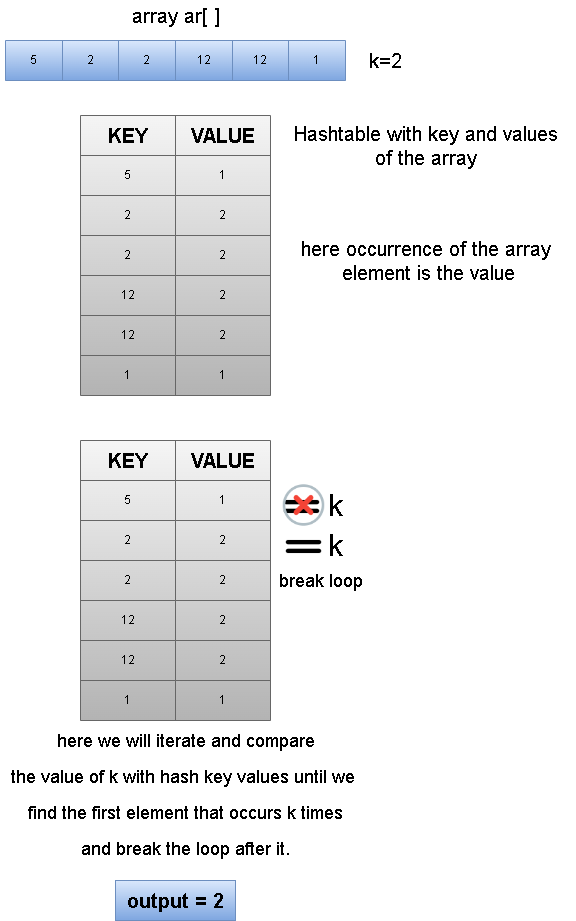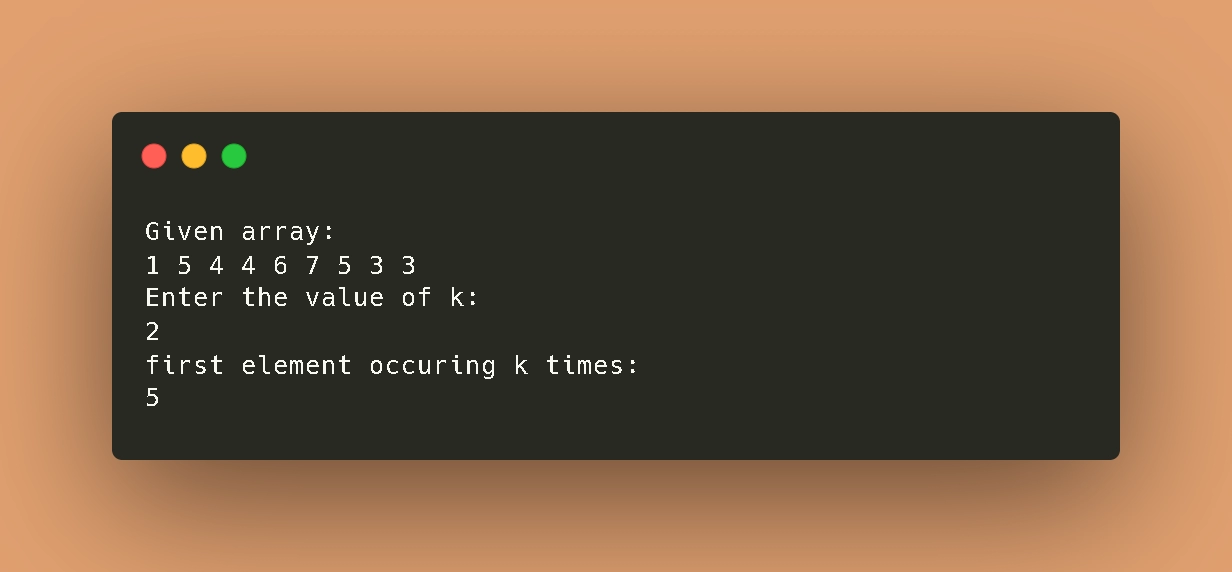New update is available. Click here to update.
Last Updated: Jun 30, 2023
Easy

# First element occurring k times in an arraydhananjay kumar
0 upvote

## Introduction

In this article, we are going to learn how we can find the first occurrence an element k times in an array with the help of hashmap.A Hash table is one of the essential data structures that uses a particular function known as a hash function that maps a given value with a key to retrieve the elements faster.

A hash table is one of the data structures used to store information. The information is composed of keys and values. The hash table can be implemented with the help of an associative array. The effectiveness of the hash function used for mapping determines how efficient the mapping process is.

### Problem Statement

The problem statement is that we need to find the first element in the array that is occurring or repeating k times the array.

### Sample Examples

Input:  [5,2,2,12,12,1] k=2

Output: 2

Explanation## ApproachIn order to find the first occurrence of the element in an array k times. We will use the hashmap approach to find the solution that is efficient. We are going to create a hashmap with a key value pair of array elements and occurrence of those elements. After that we will iterate a loop and compare the value of k with the hash key values until we find the first occurrence.

### Algorithm

• Start
• Declare an array of name ar[ ] with n elements
• Create a hashmap for the same datatype of the array
• Take input of k
• Loop n times and map the values of occurrences with their respective element
• Loop n times and compare the value of k with hash key value until we find the first element.
• Store the element in variable named result
• Display the result on screen
• End

### Implementation

C++

``````#include<bits/stdc++.h>
using namespace std;
int main()
{
int ar[] = {1,5,4,4,6,7,5,3,3};
int k,result=-1;

/* calculate the size of given array */
int size = sizeof(ar)/sizeof(ar);

/* printing the given array */
cout<<"Given array: "<<endl;
for(int i=0;i<size;i++)
{
cout<<ar[i]<<" ";
}

/* create an unordered map */
unordered_map<int,int> test;
cout<<"\nEnter the value of k: ";
cin>>k;

/* calculating the occurrence and storing as values */
for(int i=0;i<size;i++)
{
test[ar[i]]++;
}

/* comparing and finding the first occurence*/
for(int i=0;i<size;i++)
{
if(test[ar[i]]==k)
{
result = ar[i];
break;
}
}

/* printing the result  */
cout<<"first Element occuring K times: "<<result<<endl;
return 0;
}``````

Python

``````if __name__ == '__main__':
#given array
ar = [1,5,4,4,6,7,5,3,3,3]
result = -1

#calculating size of given array
size = len(ar)

#printing the array
print("Given array:",ar)

#create hashmap
test = {}

#taking input
k = int(input("Enter the value of k: "))

#mapping values
for i in range(0,size):
if(ar[i] in test.keys()):
test[ar[i]]+=1
else:
test[ar[i]] = 1

#checking the occurrence
for i in range(0,size):
if(test[ar[i]] == k):
result = ar[i]
break

print("first element occuring k times:",result)``````

Java

``````import java.util.*;
class Main {
static int firstElement(int ar[], int size, int k) {

/*unordered_map to count
occurrences of each element */
HashMap < Integer, Integer > test = new HashMap < > ();
for (int i = 0; i < size; i++) {
int a = 0;
if (test.get(ar[i]) != null) {
a = test.get(ar[i]);
}
test.put(ar[i], a + 1);
}

/* if count of element == k ,then*/
for (int i = 0; i < size; i++)

/*t is the required first element*/
{
if (test.get(ar[i]) == k) {
return ar[i];
}
}
/* no element occurs k times*/
return -1;
}

public static void main(String[] args) {
int ar[] = {1,5,4,4,6,7,5,3,3};
int size = ar.length;

/* printing the given array */
System.out.println("Given array:");
for (int i = 0; i < size; i++) {
System.out.print(ar[i] + " ");
}
System.out.println("\nEnter the value of k:");
Scanner s = new Scanner(System.in);
int k = s.nextInt();
System.out.println("first element occuring k times:");
System.out.println(firstElement(ar, size, k));
}
}``````

Input:  [1,5,4,4,6,7,5,3,3,3] k=2

Output:If the element is not present in the array that is repeating k times then the program will return -1 as an output.

### Time Complexity

Every iteration in the above programs is done in n times of the given array. Whether it is to print to or to map the values with the elements of the array it is looped in n times. So that is why the time complexity is:

O(n) here n is the size of the given array

### Space Complexity

O(n) here n is the size of the hashmap.

### What is a hashtable?

A Hash table is one of the essential data structures that uses a particular function known as a hash function that maps a given value with a key to retrieve the elements faster.

### How do we store data in a hashmap?

In hashmap data is stored in the form of the key value pair. Means every key will have some  value or data mapped to it.

### What if the element that occurs k times is not in the array?

If the element that occurs k time is not present in the array then it will return -1 as an output.

## Conclusion

In this article, we learned about how we can find a number occurring k times with the help of a hash table, and we've also looked at that in three different programming languages with the time complexity of O(n).

Must Recommended Topic -

Check out the following problems -

About DSA, competitive coding, and many more knowledgeable topics, please look into the guided paths on Coding Ninjas Studio. Also, you can enroll in our courses and check out the mock test and problems available to you. Please check out our interview experiences and interview bundle for placement preparations.Please upvote our blog to help other ninjas grow.

Happy Learning